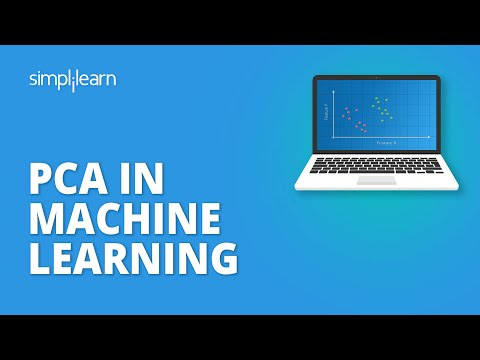While working with high-dimensional data, machine learning models often seem to overfit, and this reduces the ability to generalize past the training set examples. Hence, it is important to perform dimensionality reduction techniques before creating a model. In this article, we’ll learn the PCA in Machine Learning with a use case demonstration in Python.

## What is Principal Component Analysis?

The Principal Component Analysis is a popular unsupervised learning technique for reducing the dimensionality of data. It increases interpretability yet, at the same time, it minimizes information loss. It helps to find the most significant features in a dataset and makes the data easy for plotting in 2D and 3D. PCA helps in finding a sequence of linear combinations of variables.In the above figure, we have several points plotted on a 2-D plane. There are two principal components. PC1 is the primary principal component that explains the maximum variance in the data. PC2 is another principal component that is orthogonal to PC1.

## What is a Principal Component?

The Principal Components are a straight line that captures most of the variance of the data. They have a direction and magnitude. Principal components are orthogonal projections (perpendicular) of data onto lower-dimensional space.

Now that you have understood the basics of PCA, let’s look at the next topic on PCA in Machine Learning.

## Applications of PCA in Machine Learning• PCA is used to visualize multidimensional data.
• It is used to reduce the number of dimensions in healthcare data.
• PCA can help resize an image.
• It can be used in finance to analyze stock data and forecast returns.
• PCA helps to find patterns in the high-dimensional datasets.

## How does Principal Component Analysis Work?### 1. Normalize the data

Standardize the data before performing PCA. This will ensure that each feature has a mean = 0 and variance = 1.### 2. Build the covariance matrix

Construct a square matrix to express the correlation between two or more features in a multidimensional dataset.### 3. Find the Eigenvectors and Eigenvalues

Calculate the eigenvectors/unit vectors and eigenvalues. Eigenvalues are scalars by which we multiply the eigenvector of the covariance matrix.### 4. Sort the eigenvectors in highest to lowest order and select the number of principal components.

#### Post Graduate Program in AI and Machine Learning

In Partnership with Purdue UniversityNow that you have understood How PCA in Machine Learning works, let’s perform a hands-on demo on PCA with Python.

## PCA Demo - Classify the Type of Wine

### 1. Import the necessary libraries### 2. Load the wine dataset and display the first five rows### 3. Display the summary statistics for independent variables### 4. Boxplot to check the output labelsFrom the above box plots, you can see that some features classify the wine labels clearly, such as Alkalinity, Total Phenols, or Flavonoids.

### 5. Class separation of wine using 2 features### 6. Plot the correlation matrix### 7. Normalize the data for PCA### 8. Describe the scaled data### 9. Import the PCA module and plot the variance ratioFrom the above graph, we’ll consider the first two principal components as they together explain nearly 56% of the variance.

### 10. Transform the scaled data and put it in a dataframe### 11. Visualize the wine classes using the first two principal componentsBy applying PCA to the wine dataset, you can transform the data so that most we can capture variations in the variables with a fewer number of principal components. It is easier to distinguish the wine classes by inspecting these principal components rather than looking at the raw data.

Enhance your skill set and give a boost to your career with the Post Graduate Program in AI and Machine Learning.

## Conclusion

The principal component analysis is a widely used unsupervised learning method to perform dimensionality reduction. We hope that this article helped you understand what PCA is and the applications of PCA. You looked at the applications of PCA and how it works.

Do you have any questions related to this article on PCA in Machine Learning? If yes, then please feel free to put them in the comments sections. Our team will be happy to solve your queries. Finally, we performed a hands-on demonstration on classifying wine type by using the first two principal components.Avijeet Biswal